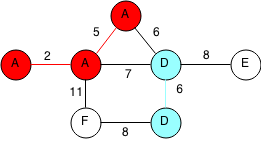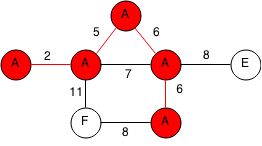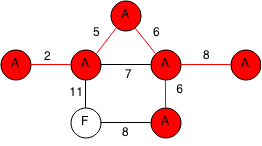## Introduction

Prerequisites: Sorting, Minimum Spanning Tree

Kruskal's algorithm finds the minimum spanning tree using connected components.

If Kruskal's algorithm is implemented efficiently using a priority queue, the runtime is O(n log n).

## Implementation

1. Uniquely label each node.
2. Take the edge with the minimum weight.
3. If the edge connects nodes A and B with different labels, all nodes with label B will be labeled with A. Otherwise, throw the edge away.
4. Repeat 2-3 until all the nodes have the same label.

### Example

We first start with each node uniquely labelled as a letter.We first start with the edge with the smallest weight of 2 which was between A and B. We add B to A's component by labelling B as A.The next edge with the smallest weight is 5 which connects to C. We add C to A's component by labelling it as A.There is a tie between edges with the smallest weight of 6 and it doesn't matter which one we take. For the purpose of this example we will take the edge between D and G. We add G to D's component be labelling it as D.Next we take the other edge with weight 6. We are connecting the D and A components, thus we can label all the nodes with D as A.The next edge with smallest weight is 7 but it does not connect two different components, thus we discard the edge. The next two edges have weights 8 and we can randomly pick the edge to E. We add E to A's component by labelling it as A.We take the other edge with weight 8 to F and add F to A's component by relabelling it as A.All the nodes are the same label and all part of the same component, thus by the algorithm, we have our minimum spanning tree.

### Code

We can implement Kruskal's algorithm efficiently by using connected components.

```class edge implements Comparable<edge> {
int weight,source,dest;
public edge(int source,int dest,int weight) {
this.source = source;
this.dest = dest;
this.weight = weight;
}
public int compareTo(edge e) {
return weight-e.weight;
}
}
public static int getParent(int parents[], int x) {
// Base Case: parent of x is itself.
if (parents[x] == x) {
return x;
}
// Set current's parent to highest parent.
parents[x] = getParent(parents, parents[x]);

// Returns parent.
return parents[x];
}

public static int Kruskal(Vector<Vector<edge>> adjList) {
int n = adjList.size();
// Array of parents of each node. Nodes with the same parent are in the same component.
int parents[] = new int[n];

// Set parents of each node to itself.
for (int i = 0; i < n; i++) {
parents[i] = i;
}

int sum = 0;
PriorityQueue<edge> edges = new PriorityQueue<edge>();

// Iterate through each node.
for (int i = 0; i < n; i++) {
// Iterate through edges of node.
for (int j = 0; j < adjList.get(i).size(); j++) {
// Add edge to priority queue.
}
}

// Iterate through all edges.
while (!edges.isEmpty()) {
// Get edge with smallest weight.
edge e = edges.poll();
// Take edge if highest parent of source and destination nodes are
// different i.e. take the edge if it connects different components
if (getParent(parents, e.source) != getParent(parents, e.dest)) {
// Set parent of source to highest parent of destination node.
parents[e.source] = getParent(parents, e.dest);

// Add edge weight to MST weight.
sum += e.weight;
}
}

// Return MST weight.
return sum;
}
```

## Exercises

1. Prove Kruskal's Algorithm works.
2. Extend Kruskals's to output the full minimum spanning tree used.